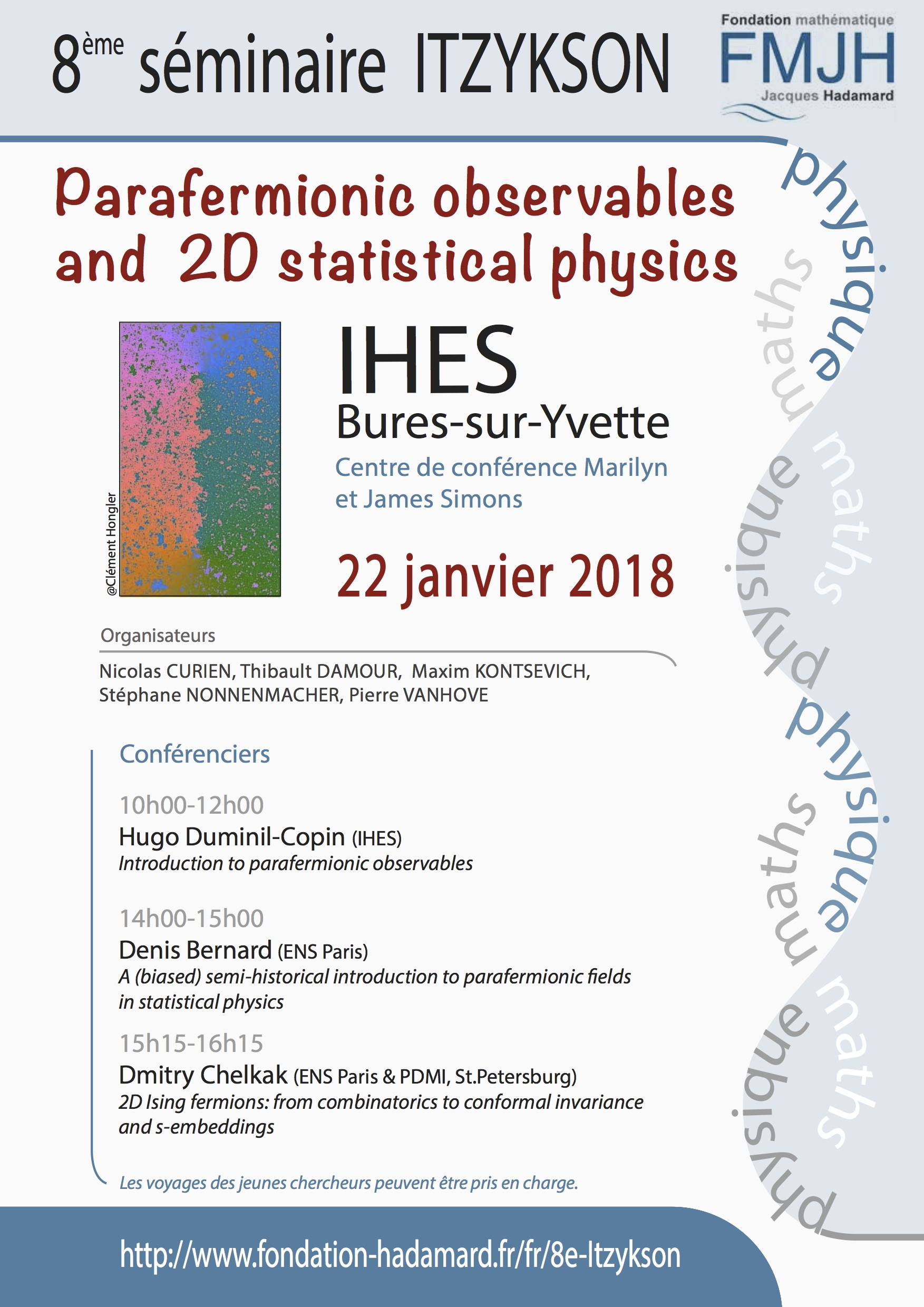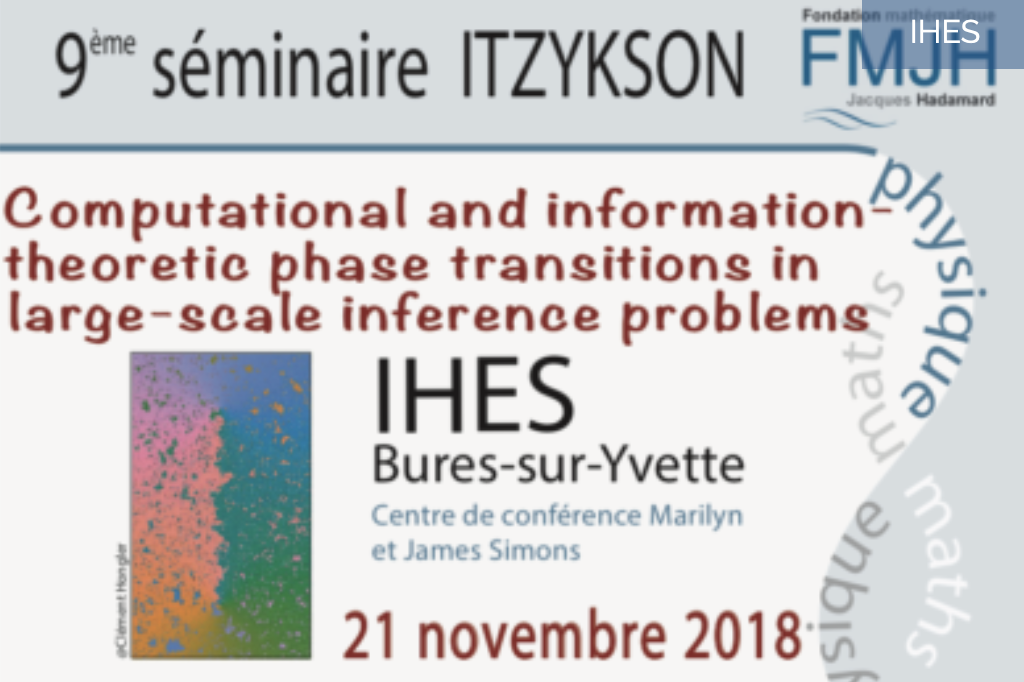## 2018 : Observables parafermioniques et physique statistique 2D

Le 8ème Séminaire Itzykson, portant sur le thème "Parafermionic observables and 2D statistical physics" s'est tenu à l'IHÉS le Lundi 22 janvier 2018.

###Programme

*************
10h-12h Hugo Duminil-Copin (IHES)

Introduction to parafermionic observables

In the early eighties, the physicists Belavin, Polyakov and Zamolodchikov postulated the conformal invariance of critical planar statistical models. This prediction enabled physicists to use Conformal Field Theory in order to formulate many conjectures on these models. From a mathematical perspective, proving rigorously the conformal invariance of a model (and properties following from it) constitutes a formidable challenge. In recent years, the connection between discrete holomorphicity and planar statistical physics led to spectacular progress in this direction. Kenyon, Chelkak and Smirnov exhibited discrete holomorphic observables in the dimer and Ising models and  roved their convergence to conformal maps in the scaling limit. These results paved the way to the rigorous proof of conformal invariance for these two models.

Other discrete observables have been proposed for a number of critical models, including self-avoiding walks and Potts models. While these observables are not exactly discrete holomorphic, their discrete contour integrals vanish, a property shared by discrete holomorphic functions. This property sheds a new light on the critical models, and we propose to discuss some of its applications. In particular, we will sketch the proof of a conjecture made by Nienhuis regarding the number of self-avoiding walks of length n on the hexagonal lattice.

**********

14h-15h: Denis Bernard (ENS Paris)

A (biased) semi-historical introduction to parafermionic fields in statistical physics

Starting from Kadanoff's and Ceva's construction, I will present an elementary introduction to different reincarnations of parafermionic observables and fields in statistical models and their relationships with braid group representations, quantum group  symmetries, integrability and holomorphicity.

**********

15h15-16h15: Dmitry Chelkak (ENS Paris & PDMI, St.Petersburg)

2D Ising fermions: from combinatorics to conformal invariance and s-embeddings

During the last decade, a number of results on conformal invariance of the critical 2D nearest-neighbor Ising model appeared, both for the small mesh size limits of correlation functions (fermions, energy densities, spins, disorders etc) and interfaces (aka domain walls) or loop ensembles arising in the model. We survey these results, which are based on discrete analysis techniques, notably on convergence theorems for solutions to some special boundary value problems for discrete holomorphic functions on rhombic lattices, and discuss the universality of the model with respect to an underlying planar graph.

## 2018 : Computational and information-theoretic phase transitions in large-scale inference problems

Le mercredi 21 novembre 2018, l’IHES a accueilli le 9ème séminaire Itzykson.Programme

* 10h30 - 12h30 : Laurent Massoulié (MSR-INRIA), Minicours (en anglais) - Video
* 12h30 : buffet
* 14h-15h : Lenka Zdeborova (IPhT, CEA): "Phase transition in regression and simple neural networks." - Video
* 15h15 - 16h15: Andrea Montanari (Stanford): "Algorithms and computational phase transitions in low-rank estimation" - Video